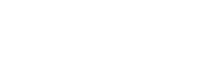#### 超越静态图的图解### 主动的交互### 交互概述

```make_dataset()
make_plot()
update()```

#### 格式化数据

1. 航班显示（在代码中称为运营商）

1. 绘图中的时间延迟范围，例如：-60 到 120 分钟

1. 默认情况下，柱状图的容器宽度是 5 分钟```def make_dataset(carrier_list, range_start = -60, range_end = 120, bin_width = 5):
# 为了确保起始点小于终点而进行检查
assert range_start < range_end, "Start must be less than end!"

by_carrier = pd.DataFrame(columns=['proportion', 'left', 'right',
'f_proportion', 'f_interval',
'name', 'color'])
range_extent = range_end - range_start

# 遍历所有运营商
for i, carrier_name in enumerate(carrier_list):
# 运营商子集
subset = flights[flights['name'] == carrier_name]
# 创建具有指定容器和范围的柱状图
arr_hist, edges = np.histogram(subset['arr_delay'],
bins = int(range_extent / bin_width),
range = [range_start, range_end])
# 将极速除以总数，得到一个比例，并创建 df
arr_df = pd.DataFrame({'proportion': arr_hist / np.sum(arr_hist),
'left': edges[:-1], 'right': edges[1:] })
# 格式化比例
arr_df['f_proportion'] = ['%0.5f' % proportion for proportion in arr_df['proportion']]
# 格式化间隔
arr_df['f_interval'] = ['%d to %d minutes' % (left, right) for left,
right in zip(arr_df['left'], arr_df['right'])]
# 为标签指定运营商
arr_df['name'] = carrier_name
# 不同颜色的运营商
arr_df['color'] = Category20_16[i]
# 添加到整个 dataframe 中
by_carrier = by_carrier.append(arr_df)
# 总体 dataframe
by_carrier = by_carrier.sort_values(['name', 'left'])

# 将 dataframe 转换为列数据源
return ColumnDataSource(by_carrier)```

(我知道这是一篇关于 Bokeh 的博客，但在你不能在没有格式化数据的情况下来生成图表，因此我使用了相应的代码来演示我的方法！)```def make_plot(src):
# 带有正确标签的空白图
p = figure(plot_width = 700, plot_height = 700,
title = 'Histogram of Arrival Delays by Carrier',
x_axis_label = 'Delay (min)', y_axis_label = 'Proportion')
# 创建柱状图的四种符号
p.quad(source = src, bottom = 0, top = 'proportion', left = 'left', right = 'right',
color = 'color', fill_alpha = 0.7, hover_fill_color = 'color', legend = 'name',
hover_fill_alpha = 1.0, line_color = 'black')
# vline 模式下的悬停工具
hover = HoverTool(tooltips=[('Carrier', '@name'),
('Delay', '@f_interval'),
('Proportion', '@f_proportion')],
mode='vline')
# Styling
p = style(p)
return p```#### 创建可交互的小部件

```from bokeh.models.widgets import CheckboxGroup
# 创建复选框可选元素，可用的载体是
# 数据中所有航空公司组成的列表
carrier_selection = CheckboxGroup(labels=available_carriers,
active = [0, 1])```CheckboxGroup 部件

Bokeh 复选框中的标签必须是字符串，但激活值需要的是整型。这意味着在在图像 ‘AirTran Airways Corporation’ 中，激活值为 0，而 ‘Alaska Airlines Inc.’ 激活值为 1。当我们想要将选中的复选框与 airlines 想匹配时，我们需要确保所选的 整型 激活值能匹配与之对应的 字符串 。我们可以使用部件的 `.labels``.active` 属性来实现。

```# 从选择值中选择航空公司的名称
[carrier_selection.labels[i] for i in carrier_selection.active]
['AirTran Airways Corporation', 'Alaska Airlines Inc.']```

```# update 函数有三个默认参数
def update(attr, old, new):
# Get the list of carriers for the graph
carriers_to_plot = [carrier_selection.labels[i] for i in
carrier_selection.active]
# 根据被选中的运营商和
# 先前定义的 make_dataset 函数来创建一个新的数据集
new_src = make_dataset(carriers_to_plot,
range_start = -60,
range_end = 120,
bin_width = 5)
# update 在 quad glpyhs 中使用的源
src.data.update(new_src.data)```

```# 将选定按钮中的更改链接到 update 函数
carrier_selection.on_change('active', update)```#### 更多控件

```# 滑动 bindwidth，对应的值就会被选中
binwidth_select = Slider(start = 1, end = 30,
step = 1, value = 5,
title = 'Delay Width (min)')
# 当值被修改时，更新绘图
binwidth_select.on_change('value', update)
# RangeSlider 用于修改柱状图上的最小最大值
range_select = RangeSlider(start = -60, end = 180, value = (-60, 120),
step = 5, title = 'Delay Range (min)')
# 当值被修改时，更新绘图
range_select.on_change('value', update)

# 用于 3 个控件的 update 函数
def update(attr, old, new):

# 查找选定的运营商
carriers_to_plot = [carrier_selection.labels[i] for i in carrier_selection.active]

# 修改 binwidth 为选定的值
bin_width = binwidth_select.value
# 范围滑块的值是一个元组（开始，结束）
range_start = range_select.value
range_end = range_select.value

# 创建新的列数据
new_src = make_dataset(carriers_to_plot,
range_start = range_start,
range_end = range_end,
bin_width = bin_width)
# 在绘图上更新数据
src.data.update(new_src.data)``````# 将绘图标题修改为匹配选择
bin_width = binwidth_select.value
p.title.text = 'Delays with %d Minute Bin Width' % bin_width```

### 把所有内容放在一起

```from bokeh.layouts import column, row, WidgetBox
from bokeh.models import Panel
from bokeh.models.widgets import Tabs
# 将控件放在单个元素中
controls = WidgetBox(carrier_selection, binwidth_select, range_select)

# 创建行布局
layout = row(controls, p)

# 使用布局来创建一个选项卡
tab = Panel(child=layout, title = 'Delay Histogram')
tabs = Tabs(tabs=[tab])```